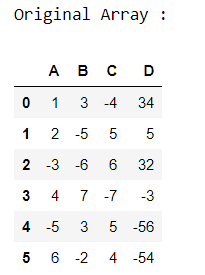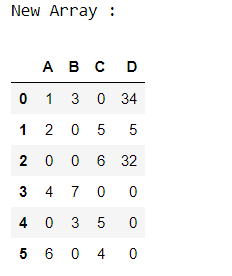Replace Negative Number by Zeros in Pandas DataFrame

• Last Updated : 05 Sep, 2020

In this article, Let’s discuss how to replace the negative numbers by zero in Pandas

Approach:

• Import pandas module.
• Create a Dataframe.
• Check the DataFrame element is less than zero, if yes then assign zero in this element.
• Display the final DataFrame

First, let’s create the dataframe.

Python3

 # importing pandas moduleimport pandas as pd  # Creating pandas DataFramedf = pd.DataFrame({"A": [1, 2, -3, 4, -5, 6],                   "B": [3, -5, -6, 7, 3, -2],                   "C": [-4, 5, 6, -7, 5, 4],                   "D": [34, 5, 32, -3, -56, -54]})  # Displaying the original DataFrameprint("Original Array : ")df

Output :Now, let’s find the negative element and replace it with zero.

Python3

 # checking the element is < 0df[df < 0] = 0print("New Array :")df

Output :My Personal Notes arrow_drop_up